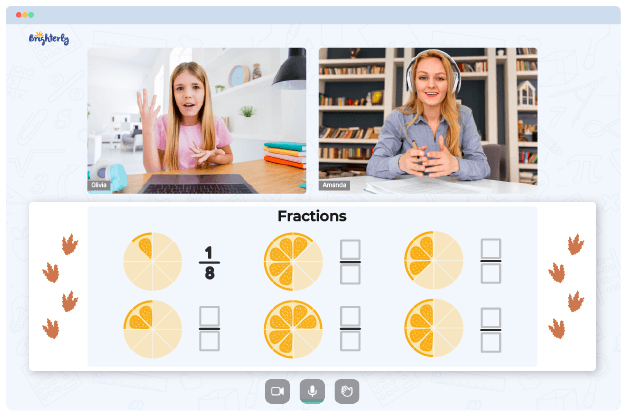# Subtracting Fractions Worksheets

Learning how to subtract fractions is no piece of cake. First, a young learner needs to have a solid grasp of the concept of subtraction. They’ll also need to understand how fractions work and the rules behind different types of fractions. Finally, they’ll combine their knowledge of these two concepts to subtract fractions effectively. Sounds like a tall order, doesn’t it? Well, it’s certainly a seamless possibility with our subtracting fractions worksheet.

## About Subtracting Fractions with Like Denominators Worksheets

The subtracting fractions with regrouping worksheet is specifically designed to test kids’ knowledge and provide them with an understanding of how the concept works. The tool contains a standard formula for subtracting two fractions. The exercises are also varied, ranging from basic ones to more difficult problems.

Math for Kids

Is Your Child Struggling With Math?
1:1 Online Math TutoringWith this worksheet, kids don’t just learn how to subtract fractions with like denominators. They go further to solve fractional problems containing unlike denominators. This way, they get a holistic and balanced understanding of the topic.

## Why Should You Get a Fraction Subtraction Worksheet?### Subtracting Fractions Worksheets PDF

Subtracting Fractions Worksheet### Subtracting Fractions Worksheets PDF

Subtracting Fractions With Like Denominators Worksheets### Subtracting Fractions Worksheets PDF

Subtracting Fractions With Regrouping Worksheet### Subtracting Fractions Worksheets PDF

Fraction Subtraction Worksheet

There are several reasons why a fraction subtraction worksheet is an absolute steal for any parent or tutor. For starters, worksheets follow an organized structure. While the exercises are varied, they follow a steady progression from easy to difficult tasks. This makes it easier for young learners to advance without getting confused. Similarly, the worksheets strike a healthy balance between comprehensiveness and gradual learning, ruling out the possibility of your kid getting overwhelmed.

### More Fractions Worksheets

Need help with Fractions?• Does your child need extra assistance with mastering the concept of fractions?
• Start learning with an online tutor.

Is your child finding it hard to understand fractions lessons? An online tutor could be of help.